Daytrading the ABCD Fibonacci Pattern (Forex Trading)

Here is an intesting video to assist with your fibonnacci trading.
It relates specifically to Forex trading.
The fibonacci tool is an especially helpfull trading indicator to assist with you with technical analysis.

Who was Fibonacci? By Mark Deaton

Leonardo Pisano, was Italian mathematician born in Pisa during the The middle Ages. He was renowned as one of the most talented mathematicians of his day. . The name Fibonacci itself was a nickname given to Leonardo. It was derived from his grandfatherâ€™s name and means son of Bonaccio.

While most attribute the Fibonacci Sequence to Leonardo, he was not responsible for discovering the sequence. In 1202 Leonardo published a book called, Liber Abaci. In it he derived a method for calculating the growth of the rabbit population.

Suppose a newly-born pair of rabbits, one male, one female, are put in a field. Rabbits are able to mate at the age of one month so that at the end of its second month a female can produce another pair of rabbits. Suppose that our rabbits never die and that the female always produces one new pair (one male, one female) every month from the second month on. The puzzle that Fibonacci posed was….

How many pairs will there be in one year?

At the end of the first month, they mate, but there is still one only 1 pair.
At the end of the second month the female produces a new pair, so now there are 2 pairs of rabbits in the field.
At the end of the third month, the original female produces a second pair, making 3 pairs in all in the field.
At the end of the fourth month, the original female has produced yet another new pair, the female born two months ago produces her first pair also, making 5 pairs.

This mathematical progression is now recognized as the Fibonacci Sequence. Starting with zero and adding one, each new number in the sequence is the sum of the previous two numbers. In our example, 0+1 = 1, 1+1=2, 1+2=3, 2+3=5, and so on.

The sequence of numbers looks like this: 0, 1, 1, 2, 3, 5, 8, 13, 21, 34, 55, 89, 144, 233, to infinity. From this sequence you can easily reason that at the end of one year there would be 233 pairs of rabbits.

This sequence has repeatedly appeared in popular culture from architecture to music to television. While the series is a powerful tool, the analysis of one number with the number up to four places to the right. The first three are shown below.

While some are not exact, if you repeat this mathematical analysis through multiple sets of data, you will see we arrive at some well known and fairly consistent ratios.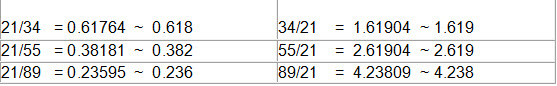The dimensional properties adhering to the 1.618 ratio occur throughout nature and the ratio is most referred to as The Golden Ratio. The uncurling of a fern and the patterns found on various mollusk shells are commonly cited examples of this ratio.

This number, when added to 0.618, equals 1.

These ratios have been used for over a hundred years in the financial markets by the likes of W.D. Gann and Ralph Nelson Elliot. Up until the late 90s the tracking and use of these numbers were a manual process.

With the proliferation of real-time charting and data, software that automatically calculated and displayed these levels brought Fibonacci into the financial mainstream.

Fibonacci as a Technical Analysis Tool
While there have been countless books and articles written on the use of Fibonacci in technical analysis, the basics are simple. Fibonacci Trading is an art form anyone can master with a little practice.

On the price scale, these ratios, and several others related to the Fibonacci sequence, often indicate levels at which strong resistance and support will be found. Many times, markets tend to reverse right at levels that coincide with the Fibonacci ratios. On the time scale Fibonacci ratios are one method of identifying potential market turning points. When Fibonacci levels of price and time coincide you have high probability entry points.

Author

Mark Deaton
www.fibonaccigenius.com

Excellent article about Neuro Net Trading Robot

Hi Guys

I just have to let you know about an excellent article.

It talks of the Leo Trader Pro.

The never before seen technology used to programme this unique expert advisor makes it a must have.

And…theres a bonus giveaway too!

To find out more, while there is still time, head straight over to the article here.

For another great and 100% free offer you can check this out!

Fibonacci Summary by Mark Deaton

Fibonacci ratios are one of the most commonly used techniques in technical analysis of the financial markets. If you are already a successful trader or a trader just starting out, Fibonacci Retracements provide valuable insight and triggers on where high probability change will happen.

The Fibonacci Retracement is likely the most common of all Fibonacci related tools. While there are many variations of the ratio set, I think simple is better.
23.6% — The shallowest of the retracements. In very strong trending markets price typically quickly bounces in the area of this ratio.
38.2%— This is the first line of defense of the current trend. Breaking this level starts to erode the underlying trend.
50% — the neutral point of any retracement. This is the critical tipping point.
61.8% — retracing to this typically signals a breakdown in the trend.
100% — Matching the initial move
138.2%
161.8%
200%
Within this set, 38.2%, 50% and 61.8% I find quite reliable. I use the others as more confirmation and as part of clusters.

The Fibonacci Extension is less common but just as powerful when correctly applied. Used as part of a money management strategy and as profit target projection tool, Fibonacci Extensions provide guidance on where price will potentially stall or change direction.

Used in combination, these two ratio sets provide very tradable indicators of opportunity. If you then combine Fibonacci with other indicators like oscillators and moving averages, you can quickly identify high potential risk/reward opportunities. While not perfect, they are one of the best tools available.

Author
Mark Deaton
http://www.fibonaccigenius.com

Fibonacci Clustering- by Mark Deaton

In our previous examples we have seen how both Fibonacci Retracements and Fibonacci Extensions can be powerful tools on their own. Often, I use multiple Fibonacci Retracements to determine entry and exit points. And then also combine Fibonacci Extensions.

Letâ€™s look at an example where using multiple Fibonacci Retracements prove very useful. In Figure 13 we see the dramatic fall of the USDCAD that we looked at from a different perspective in Figures 4, 5 & 6.
Fibonacci clustering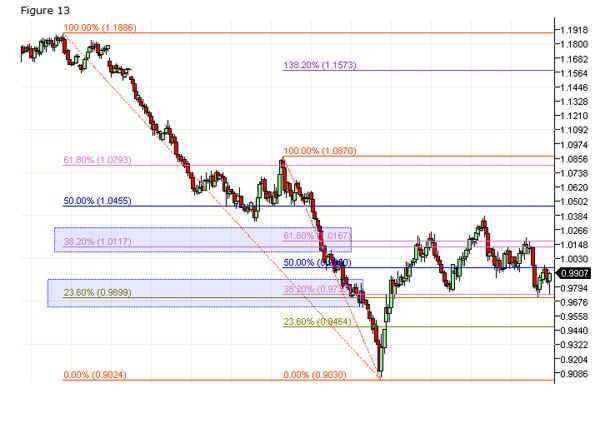In the figure above I have added two Fibonacci Retracements. Take special note to the two areas highlighted. These areas identify clusters of Fibonacci levels.

The bounce off the bottom blew directly though the first cluster of 23.6% and 38.2%. In subsequent retracements, this level became support and you could have safely used it as a very low risk entry point.

Additionally, the 38.2% and 61.8% cluster became resistance. In two of three cases you could have used this information for low risk short side entry points.

The key to remember in this example: You had all this information immediately after the swing low was established. Therefore knowing these levels this early in the swing allows these levels to be predictive
Now letâ€™s add one more Fibonacci Retracement.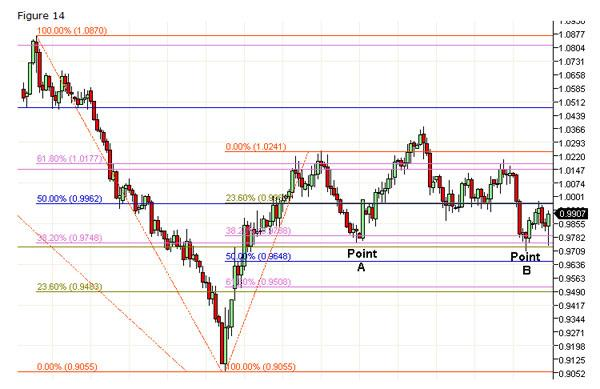In the figure above I have added a third Fibonacci Retracement.

For the patient trader, the third set Fibonacci Retracement levels provides more confidence that both Point A and Point B are high quality and low risk entry points from the long side.

After practicing this method for a while, you will find it common for you charts to have multiple Fibonacci Retracements. Learning to create and read Fibonacci Retracement clusters is a powerful and valuable tool for your Fibonacci Toolset.

You can also cluster multiple Fibonacci Extensions. In Figure 15 you see this in action.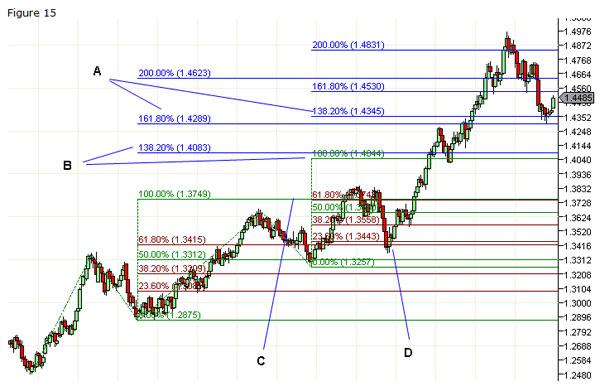In this chart you see two different sets of Fibonacci Extensions applied. As you can see there are four, two level groups. Each of these groups represents low risk entry points. Ideally, you would want to initiate the position somewhere between the two levels. Additionally, with this type of setup, you can almost trade from pair to pair.

As you can see by the previous two examples, it would be very easy to draw countless retracements and extensions on virtually any chart. It really depends on the chart and the price action.

In Figure 7 we looked at the Bottoming of the GBPUSD using one basic Fibonacci Retracement. Letâ€™s revisit GBPUSD on a bigger scale and add to our thesis.

In our next chart I have added a larger Fibonacci Retracement that encapsulates the entire top to bottom move and I have added a Fibonacci extension from the high and low swing points within the retracement price action. These additions are shown on our chart below.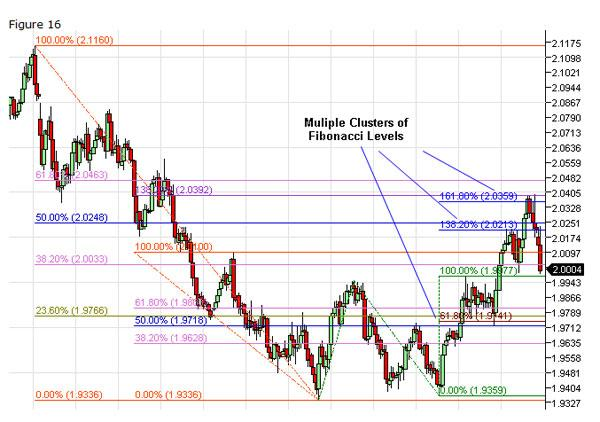Take special notice of the Fibonacci clusters highlighted. Each of these clusters served as either support or resistance points. And each created a tradable opportunity.

Author

Mark Deaton
www.fibonaccigenius.com

Fibonacci-Extensions by Mark Deaton

Picking a high potential entry point is half the battle. How do we know what kind of move price is expected to make? Projecting price movement beyond the swing points is the answer everyone seeks.

In our first example we entered a long position on the 23.6% retracement from the swing high. How far can we expect the position to run? In Example 3, we identified 3 potential entry points. Now it is time to figure out possible exit points.

Fibonacci Extensions take into account three price points. A swing high and swing low, plus the swing point where price reversed from a retracement.

Like Fibonacci Retracements, I use exactly the same set of Fibonacci levels when using Fibonacci Extensions.

Letâ€™s go back and look at our first example. We see in figure 3 the trade could have been a big winner, but how would we figure out potential exit points? Fibonacci Extensions!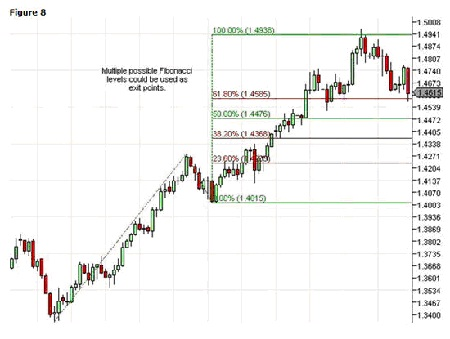I find Fibonacci Extensions the most useful as a money management tool.
The last thing we want to do is turn a winner into a loser. As price moved above the 23.6% level, I would start to bring my stop loss up in trail. Accordingly, I would continue to raise my stop as price increased.

The level of risk is entirely up to your trading plan, but tools like Fibonacci Extensions help identify key levels from which stops can be placed.

You can see in the next chart, Figure 9, that after price penetrated the 100% Fibonacci Extension level, we entered into a period of consolidation. While during the consolidation there were obvious entry points, however, remaining in the initial trade would have proven to be frustrating.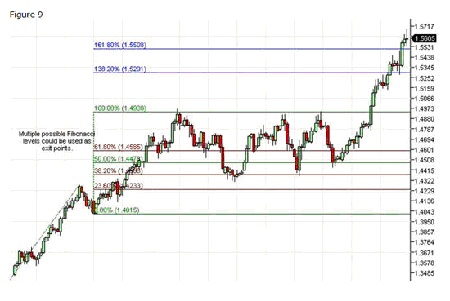In Figure 7 we found the GBPUSD creating a bottom and breaking out to the upside. We also identified 3 potential entry points. Letâ€™s look at each of the entry points and use Fibonacci Extensions to project profit targets.

In the first trade we entered the market thinking that a swing low was in place off the 38.2% Fibonacci Retracement level.
You can see in Figure 10, while price did bounce to the 23.6% Fibonacci Extension level. It was enough to make a profit, but it was not a major winner.

This is a trade that once you did not see a continuation through the 23.8% level, you could have take that as a cue to exit the position. Or at the very least move you stop up to maintain some level of profit.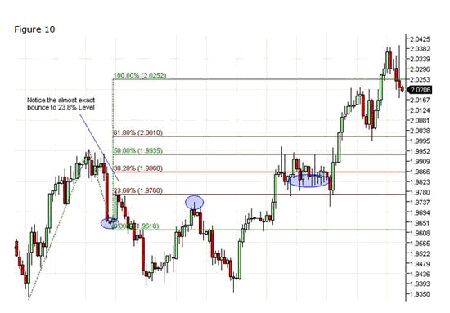Moving on to the second entry point, we sold short at the 50% Fibonacci Retracement level.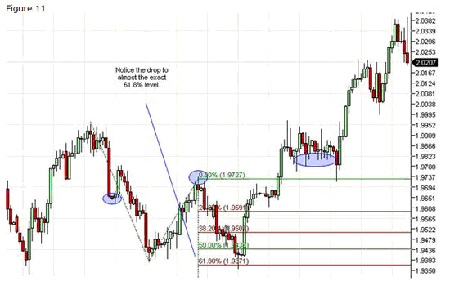This was obviously a solid entry point. On the way down you can clearly see opportunity to tighten up your stop loss values. There are many ways to manage a trade like this without being overly tight with your stops.

In fact some would have taken profit at the 23.6% Fibonacci Extension level. I believe that taking profit at any of the levels would have been a prudent strategy.

Now letâ€™s look at the final entry point from our Figure 7 example.

This trade was by far the most troubling. Even the correct entry could have been painful. However, if you did stick it out, there were multiple exit points based on the Fibonacci Extensions.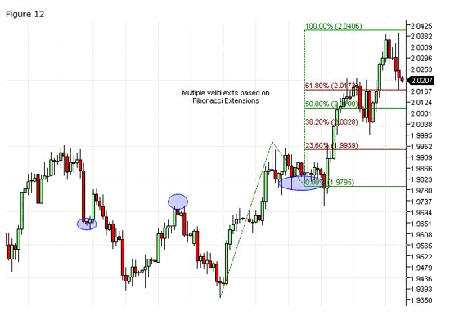Author:

Mark Deaton
www.fibonaccigenius.com

Fibonacci Analysis by Mark Deaton

Fibonacci Analysis is the single best way to predict price action.

Through careful assesment of a longer time frame then the one you are trading you can quickly identify where price will go to next and where it will likely encounter resistance and reverse.

The proper way to implement Fibonacci analysis is by means of Fibonacci retracements and extensions. These terms describe the two means of forecasting price action horizontally speaking. Truthfully this is the only way I use Fibonacci anyway.

Horizontally, meaning horizontal lines across your chart. A retracement is when price starts at a low or high then makes a new low or high and then begins to correct. The corrective wave is the retracement you are measuring and this type of Fibonacci analysis is measuring the move back to the start.
In other words if price makes it all the way back we would say that price has retraced 100%.

If however price makes it just halfway back we would say price has retraced how much?

Thatâ€™s right 50%.

Lets look at a 50% retracement… In the image below price starts at 100% comes down to 0% and begins to rise – retracing its way back to 0%. Understand we didn’t lay down the Fibonacci levels until AFTER we reached 0% and began to retrace then and only then do we do our Fibonacci Analysis. Notice at the 50% level price encounters serious resistance and fails to penetrate.Your Fibonacci analysis should consist of learning how to properly identify Fibonacci retracements as well as Fibonacci extensions and thats it. These levels are your key to forecasting high probability price reversals and continuations.

When you begin to analyze your chart using Fibonacci analysis understand that what you are looking for is simple confluence. The heart of Fibonacci is really nothing more than support and resistance levels that have demonstrated hesitation or reversals at these levels you are looking at now.

The more you see in the past that concides with your current analysis the more you will realize that your analysis is accurate. If the only time price is hitting a Fibonacci line is right now, then your analysis is likely innacurate.

So when you are doing your Fibonacci analysis make sure that the area you have identified as a retracement level is also a level that has been tested in the past and proven to be an important level.

Your job is to identify high odds places on the chart so you need this kind of agreement otherwise you may as well flip coins.Author:
Mark Deaton
www.Fibonaccigenius.com

Fibonacci Retracements

The Fibonacci Retracement is probably the most heavily used Fibonacci tool in the toolset. You will find Fibonacci Retracements as a solid tool in identifying key support and resistance areas.

If prices have fallen from a recent swing high down to a swing low, the expectation is that price should retrace distance, high to low, by a ratio of the Fibonacci sequence.

You can use Fibonacci retracements and extension from a tick chart through a daily, monthly and weekly. Literally any time frame.
It is important to note, the larger price move from swing high to swing low, the more accurate the retracement projections. Identification and selection of the correct swing points are keys to success.

While there are many variations of the ratio set, simple is better, lets focus on four major retracement levels.
â€¢ 23.6% — The shallowest of the retracements. In very strong trending markets price typically quickly bounces in the area of this ratio.
â€¢ 38.2% — This is the first line of defense of the current trend. Breaking this level starts to erode the underlying trend.
â€¢ 50% — The neutral point of any retracement. This is the critical tipping point.
â€¢ 61.8% — retracing to this typically signals a breakdown in the trend.
â€¢ 100% — Matching the move

In this section we will also show examples of how potential opportunities form when price retraces beyond 100% by following another set of Fibonacci ratios:
â€¢ 138.2%
â€¢ 161.8%
â€¢ 200%

Notice in each case we have simply added 100% to the standard ratio set. I use this set of retracements on a daily basis, from 23.6% all the way to 200% and sometimes 300% For my style of trading I find 38.2%, 50% and 61.8% quite reliable.I use the other primarily as confirmation levels.

So lets take a look at some examples of Fibonacci Retracements in use.

Example 1:

Take the example below. The EUR/USD had risen from 1.3360 to 1.4278. The next day the EURUSD failed to make a new high and the potential swing point was in place. So I using swing points I placed a Fibonacci retracement on my chart.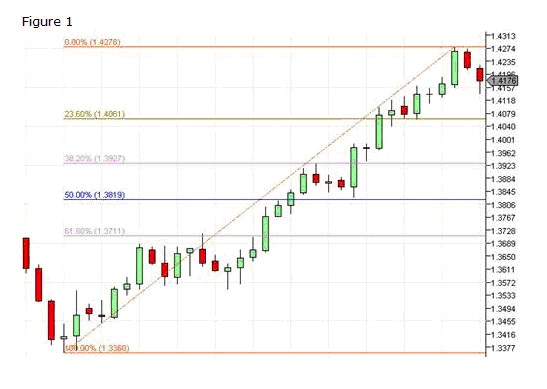The trend was obviously very strong and the first retracement to the 23.6% level was met with a violent change in direction. You can see the dip below the 23.6% level and the sudden reversal. While there are multiple entry methods, the most conservative would be to wait until the level is penetrated and price establishes itself above that level and enter on the open of the next bar as shown.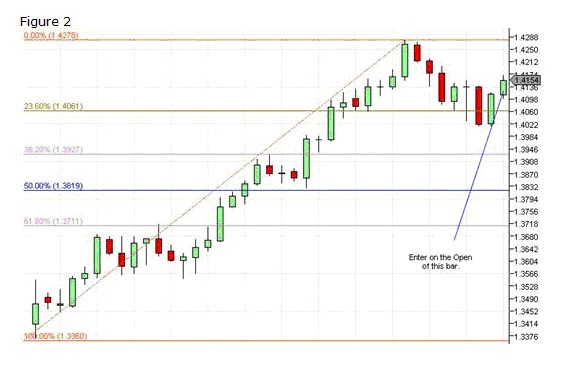With the right money management, you can see in this example this could have been a serious winner.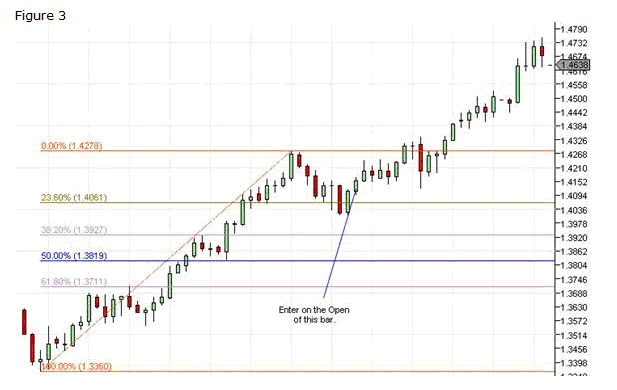Once you understand the method you can find countless examples. Every market, FOREX, Equities and Futures each exhibit these patterns to some degree.

Example 2:
Lets look at another example using the USDCAD. You can see in this example there are multiple entry points for both trend and countertrend trades.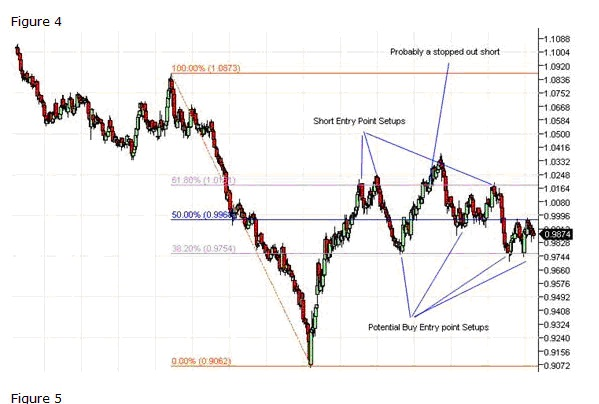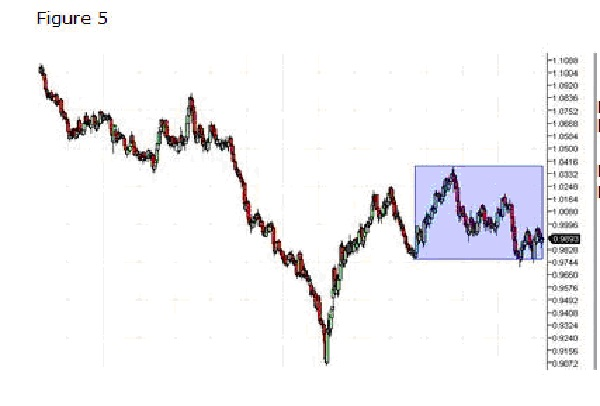Lets zoom in and look at the area highlighted in blue. Fibonacci Ratios work on virtually any size price swing.
The chart below shows the Fibonacci Retracement applied to the smaller price swing.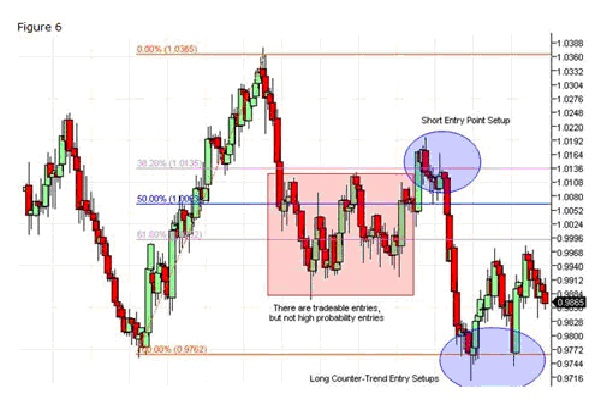The blue ellipses show the high potential entry points. Notice, in each of these cases you could have entered the market with a relatively tight stop loss with high reward potential.

Ok, we have shown some examples of well behaved price action. What happens if price retraces 100%? How far can it go beyond this point? Fibonacci ratios provide some clues to answering this question and finding low risk entry points.

Example 3:
The example below shows the GBPUSD making a bottom and bouncing back. And multiple entry points from the same set Fibonacci Retracement levels.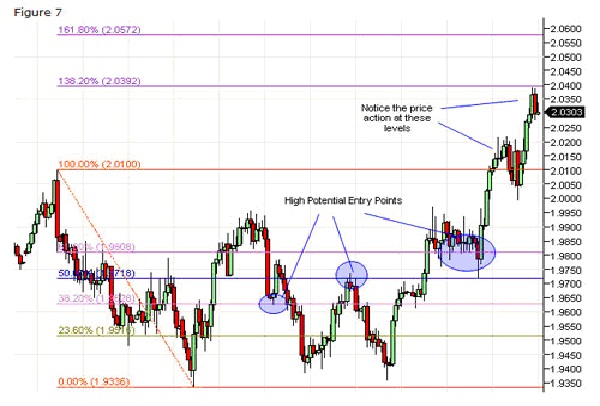Of note are the high potential entry points at 38.2%, 50% and 61.8%. Each of these could have been entry points with solid profit potential. However, notice after the initial breakout above 100%, there were other opportunities to get in the trade. Ultimately price jumped to the 138% point before backtracking.

This example shows yet another way to use Fibonacci Retracements. This example shows why it is valuable to identify potential levels above and beyond the initial 100% retracement.

Retracements are the cornerstone of Fibonacci theory as it applies to the financial markets. Hopefully these examples have provided guidance from which to draw your own retracements and expand your trading toolset.

To recap, while there are other retracement values, my defaults Fibonacci Retracements always include: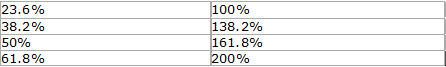You can never tell when price action it going well beyond the 100% level.

Author:

Mark Deaton
www.Fibonaccigenius.com

Posted in Uncategorized | 1 Reply

Fibonacci Trading by Mark Deaton

Fibonacci Trading is not difficult. If you are new to using Fibonacci tools then look around this site and be sure to download our free manual and Fibonacci trading system.

The system is simply a tool that you can use to begin to learn just how you can use Fibonacci trading tactics in your overall trading strategy.

Fibonacci trading simply involves measuring swing highs and lows and attempting to forecast price reversals and continuations.

Fibonacci trading concepts can get complicated, the story and science behind it can get fascinating and even a bit overwhelming. If you want to learn about the theories and science behind Fibonacci trading concepts you are at the wrong web site.

If however you want step by step instructions on how you can begin to apply the trading principles using Fibonacci then you have the arrived.

Fibonacci Trading In PracticeWhen price makes its way in any direction up or down a Fibonacci retracement measures its mark back to where it started. So lets say price started at 40.00 and then went to 50. Thatâ€™s a 10.00 move right?

Well generally speaking after a move like that there tends to be a degree of profit taking from traders. People are going to start selling off and cashing in on the move.

These moves and profit taking scenarios are what causes the markets to move up and down. Generally in a bull market you will see a large move up, a retracement profit taking period and then a new thrust up once again.
The market stays bullish as long as the profit taking doesn’t meet or exceed a 100% retracement.
For example a \$10.00 move up in a week, then a \$5.00 retracement the next is a 50% retracement.
If price moves up \$10.00 again then we are making bullish progress. \$40 to \$50 is a \$10 dollar move. A pullback or retracement to \$45 is a 50% retracement. A new \$10 dollar move up is a target of \$55.

So in time we are making our way up in a fashion that is as old as time itself.
These Fibonacci trading principles are called retracements.

Even if price were making 76% retracements we are still making bullish progress. Weak progress but bullish none-the-less.

In a super strong bull market a retracement as little as 23.6% is commonplace.

So you see the degree of retracement can tell you much about market strength.

Lastly anything beyond a 100% retracement is called a Fibonacci extension.

Author:
Mark Deaton
www.Fibonaccigenius.com